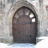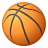# Calculating the n-th percentile of all point features that fall within a polygon

9359
7
09-27-2011 02:27 AMNew Contributor
Hi everyone,

I'm new to ArcGIS (GIS in general) and the forums so I appologise if this is not the correct place for this question. Please move it if this is not the case.

The problem I'm having is stated in the title. I have a feature class with points containing several attributes. I also have a class containing polygons that represent neighbourhoods. Now I need a way to calculate the n-th percentile of all points that fall within a certain neighbourhood and also add this percentile to the class. With my limited knowledge of ArcMAP I have come up with the following approach:

1: Join the points to the polygons
2: Add a new field to the attribute table
3: Calculate percentiles with the field calculator using a VB script.

The problem with this approach is that I don't know VB. I do have it written down in pseudo code though. My question really is, if there is another way to accomplish this? Searching the support resources didn't do me any good. I also couldn't find a VB script. If anyone could point me in the right direction it would be very much appreciated.

Regards,

Hayvic
Tags (3)
7 RepliesbyMVP Esteemed Contributor
Seems to me you could do this in ModelBuilder with the standard tools -- using the Calculate Value tool to run a little python script to calc the percentiles using an update cursor.  Any takers?New Contributor
Thanks for pointing me in that direction. I was meaning to learn Python anyway. Hopefully it's not too different from Java.

EDIT: On a related note, can anyone point me in the direction of a good online python tutorial?New Contributor
Hi Hayvic,
This is a good simple source https://www.e-education.psu.edu/geog485/node/17
If you are familiar with other programming languages you could use this one too  http://diveintopython.org/

I agree that using model builder is enough for your problem.New Contributor
EDIT: Solved it myself. It was a matter of two parenthesis. It should read List.sort() instead of List.sort. Oh programming, how I've missed you. And the hardest part is yet to come...

Hi everyone,

A small update: I've created a small script to calculate percentiles using IDLE.

```##This script calculates the n-th percentile of a list of numbers that can be read from
## a shape file or table

#P is the percentile
P = 0.3

import math
import arcgisscripting
gp = arcgisscripting.create()

#The location of the file to be read
gp.Workspace = "path to the folder or gdb"
#name of the file
cur = gp.SearchCursor("the name of the shape file or table")

List = []
row = cur.Next()
while row <> None:
List.append(row.VerticalVelN)
row = cur.next()

List.sort                               #Sort the list

#Excel method to determine the percentile

n = P * (len(List)-1)+1  #rank

if n == 1:
N = List[n-1]
elif n == len(List):
N = List[len(N)-1]
else:
rank = math.modf(n)
d = rank
k = int(rank)
N = List[k-1] + d * (List - List[k-1])

print N```

I've used the Excel method as I figured the results would be easy to verify. There seems to be a small problem however. If I make a list of random numbers myself, for example

` List = [15, 20, 35, 40, 50,20.1,15.2,55,60]`

I get the same result as Excel. However, if I use the above code to read a shape file of 5551 data points there is a slight difference, e.g. the 30th percentile is -2.7 instead of -2.5 given by Excel. I figured there could be 3 possibilities:

1: The reading of the shape file is not correct.
2: There's a bug in the algorithm that is only apparant on large data sets
3: The small difference is acceptable

I ruled out number one by simply printing the list and verifying that the entries correspond. What do you guys think?

Thanks!New Contributor
Well, after working on it on and off for the last week I finally managed to produce a working code. I think it's the most inelegant code ever written and it's extremely slow, but it works! Somewhere along the way I forgot I had set out to make it work within the ArcMAP environment so I ended up with a script that works on his own, albeit with cursor methods provided by arcgis scripting. It shouldn't be too hard to convert it to something that will work with calculate value but frankly, I'm done with it 😄

```
##This script calculates the n-th percentile of x number of point objects that fall within y number of polygon objects##

#workspace is the name of the root folder or gdb where the shape file or table can be found
workspace = "...."
#bestand is the name of the shape or table to be read
bestand = "...."
#the name of the field that contains the values for which you want to know the percentile
name = "...."
#A unique ID field that identifies the polygon object
id = "...."

P = 0.3                                 #P is the percentile

import math
import arcgisscripting
gp = arcgisscripting.create()

gp.Workspace = workspace
cur = gp.SearchCursor(bestand)

List = []
List2= []
row = cur.Next()
while row <> None:
List.append(row.name)
List2.append(row.id)
row = cur.next()

List3 = [None] * len(List)              #This list is used to store the calculated percentiles later on
M = [List, List2, List3]

#The list is sorted by ID
indices = range(len(List))
indices.sort(key = M.__getitem__)
for i, sublist in enumerate(M):
M = [sublist for j in indices]

#Keeps track of the row numbers for wich the list jumps to the next unique ID
grens = []
grens.append(0)

p = 0
count = 0
while p<=48 and count<=(len(List)-2): #48 is a hardcoded number of unique polygon objects

#This block counts the number of instances of all unique polygon ID's.
temp = M[count]
while M[count]==temp:
if count == len(List)-1: break
else: count += 1
if count == len(List)-1: grens.append(count)
else: grens.append(count-1)

#Here a subpart of the list is sorted on ascending order of point object values
if count == len(List)-1: M[grens
+1:count+1]=sorted(M[grens
+1:count+1])
elif p == 0: M[grens
:count]=sorted(M[grens
:count])
else: M[grens
+1:count]=sorted(M[grens
+1:count])

#Excel method for calculating the percentile

#rank
if p == 0 or count == len(List)-1: n = P * (count-grens
-1)+1
else: n = P * (count-grens
-2)+1

if n == 1:
n = int(n)
N = M[n-1]
elif n == count-grens
:
N = M[count-grens
-1]
else:
rank = math.modf(n)
d = rank
if p == 0: k = int(rank) + grens

else: k = int(rank) + grens
+ 1
N = M[k-1] + d * (M - M[k-1])

#The calculated percentile is saved in the list
if p==0: k=grens

else: k=grens
+1
while k<=count:
M=N
k += 1
p += 1

#The calculated percentiles should now be written to the file
i = 0
while i < len(List2):
where = "id=" + str(M)
cur = gp.UpdateCursor(bestand, where)
row = cur.Next()
while row:
row.SetValue("percentiel03", M)           #percentiel03 is the name of the new field
cur.UpdateRow(row)
row = cur.Next()
i += 1

```

Few notes:

1: There is one hard coded value which is the number of unique polygon objects. This shouldn't be too hard to fix, but I have already spent too much time on this.
2: The writing process is painfully slow. It might be spead up by using the following piece of code instead:

```j = 0
i = 0
while j <=len(grens)
while i < len(List2)
where = "id=" + str(M[grens+1])
cur = gp.UpdateCursor(bestand, where)
row = cur.Next()
while row:
row.SetValue("percentiel03", M)           #percentiel03 is the name of the new field
cur.UpdateRow(row)
row = cur.Next()
i += 1
j += 1
```

This is the more logical procedure as it skips to the next unique ID instead of going through the entire list. However I haven't tested this

Feel free to use, modify or discard the code. I am also very muc open to critique on my coding habits.

Thanks for the help everyone.

Regards,

HayvicNew Contributor
Hi,

Is it possible to calculate the percentile rank in a table?
I have chemical analysis result in a table and i want to calculate the percentile rank of each point (in a new column...). I can do it on Microsoft excel but i'm looking for a way to do it directly on Arcmap...(or model builder)byMVP Esteemed Contributor
This function I found in stack-overflow would do the trick.

An approach to doing this in ArcGIS would be

2. run the function on the list to get the percentiles
3. load the values and percentiles into a dictionary with zip
4. add a percentile field to the table
5. populate the percentile field values using an update cursor

If you don't want to create a script tool, you could put the python code wrapped as a function with the input table as its argument) in ModelBuilder's Calculate Value tool.

```## {{{ http://code.activestate.com/recipes/511478/ (r1)
import math
import functools

def percentile(N, percent, key=lambda x:x):
"""
Find the percentile of a list of values.

@parameter N - is a list of values. Note N MUST BE already sorted.
@parameter percent - a float value from 0.0 to 1.0.
@parameter key - optional key function to compute value from each element of N.

@return - the percentile of the values
"""
if not N:
return None
k = (len(N)-1) * percent
f = math.floor(k)
c = math.ceil(k)
if f == c:
return key(N[int(k)])
d0 = key(N[int(f)]) * (c-k)
d1 = key(N[int(c)]) * (k-f)
return d0+d1

# median is 50th percentile.
median = functools.partial(percentile, percent=0.5)
## end of http://code.activestate.com/recipes/511478/ }}}```

http://stackoverflow.com/questions/2374640/how-do-i-calculate-percentiles-with-python-numpy

(Note there are some other recipes toward the end of that thread that may be more what you want.)